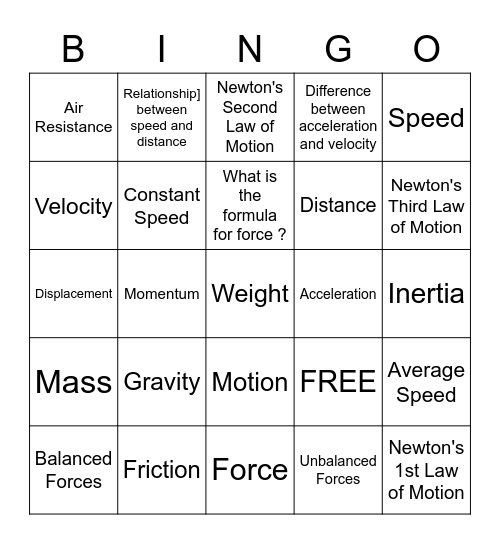# 8th Motion and ForcesThis bingo card has 25 words: Gravity, Force, What is the formula for force ?, Balanced Forces, Speed, Air Resistance, Velocity, Relationship] between speed and distance, Acceleration, Motion, Distance, Displacement, FREE, Average Speed, Constant Speed, Newton's 1st Law of Motion, Inertia, Newton's Second Law of Motion, Newton's Third Law of Motion, Momentum, Weight, Friction, Mass, Difference between acceleration and velocity and Unbalanced Forces.

## Play Online